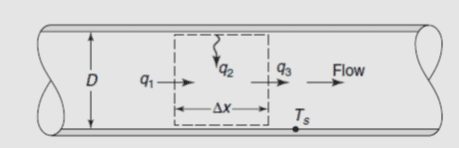# Conceptual Heat X-fer Question (Steady state, liquid changing temp as it flows)

## Homework Statement

Not hw.. My teacher wants us to understand this as it is important for reactor design.

A liquid is flowing through a tube of diameter D. The inner wall of the tube is maintained at a constant temperature of Ts. The liquid enters at a temperature of Tw1and leaves at a temperature of Tw2. The heat transfer coefficient for the liquid under the conditions of flow is h. The length of the tube is L. Assume steady state. Show that the rate at which heat is transferred from the wall to the flowing liquid is given by-

## Homework Equations

q=h (π D L)((Ts−Tw1)−(Ts−Tw2))/ln((TS−Tw1)(TS−Tw2))

## The Attempt at a Solution

I am trying to understand why the above equation is right, the only thing I can't seem to get is the ln of the bottom term, I understand the numerator, or atleast I think I do.

Last edited:

Chestermiller
Mentor
If you don't understand the denominator then you don't understand the numerator.

The starting equation for this derivation is:
$$WC_p(T(x+\Delta x)-T(x))=\pi D \Delta x h(T_s-T(x))$$
where W is the mass rate of flow and Cp is the heat capacity. Do you understand where this equation comes from?
Divide both sides of the equation by delta x, and take the limit as delta x approaches zero. What differential equation do you get?

Chet

This is embarrassing but I realized that I wasn't using an energy balance while trying to solve for the heats....

Thank you!